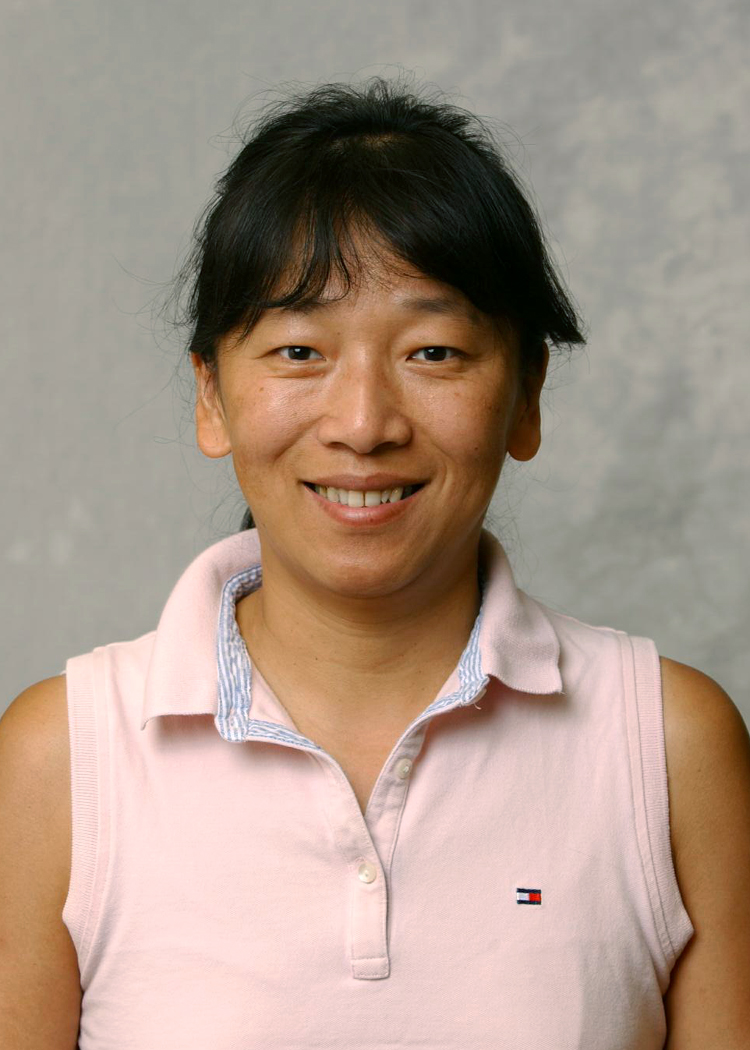# Dr. Min ChenProfessor of Mathematics
• 765 49-41964
• MATH 418
chen45@purdue.edu
###### Research Interest(s):
numerical analysis, partial differential equations, scientific computing

#### Publications listed in MathSciNet

•  Min Chen and Gérard Iooss. Periodic wave patterns of two-dimensional Boussinesq systems. Eur. J. Mech. B Fluids, 25(4):393-405, 2006.
•  A. A. Alazman, J. P. Albert, J. L. Bona, M. Chen, and J. Wu. Comparisons between the BBM equation and a Boussinesq system. Adv. Differential Equations, 11(2):121-166, 2006.
•  Min Chen and Gérard Iooss. Standing waves for a two-way model system for water waves. Eur. J. Mech. B Fluids, 24(1):113-124, 2005.
•  Min Chen, D. J. Kaup, and Boris A. Malomed. Three-wave solitons and continuous waves in media with competing quadratic and cubic nonlinearities. Phys. Rev. E (3), 69(5):056605, 17, 2004.
•  J. L. Bona, M. Chen, and J.-C. Saut. Boussinesq equations and other systems for small-amplitude long waves in nonlinear dispersive media. II. The nonlinear theory. Nonlinearity, 17(3):925-952, 2004.
•  M. Chen. Equations for bi-directional waves over an uneven bottom. Math. Comput. Simulation, 62(1-2):3-9, 2003. Nonlinear waves: computation and theory, II (Athens, GA, 2001).
•  J. L. Bona, M. Chen, and J.-C. Saut. Boussinesq equations and other systems for small-amplitude long waves in nonlinear dispersive media. I. Derivation and linear theory. J. Nonlinear Sci., 12(4):283-318, 2002.
•  Min Chen. A plethora of multi-pulsed solutions for a Boussinesq system. In Mathematical and numerical aspects of wave propagation (Santiago de Compostela, 2000), pages 274-279. SIAM, Philadelphia, PA, 2000.
•  Min Chen. Solitary-wave and multi-pulsed traveling-wave solutions of Boussinesq systems. Appl. Anal., 75(1-2):213-240, 2000.
•  Rolf Bronstering and Min Chen. Bifurcations of finite difference schemes and their approximate inertial forms. RAIRO Modél. Math. Anal. Numér., 32(6):715-728, 1998.
•  M. Chen. Exact solutions of various Boussinesq systems. Appl. Math. Lett., 11(5):45-49, 1998.
•  Min Chen. Primary results on simulation of a new kind traveling wave of permanent form. In Mathematical and numerical aspects of wave propagation (Golden, CO, 1998), pages 209-213. SIAM, Philadelphia, PA, 1998.
•  Min Chen. Exact traveling-wave solutions to bidirectional wave equations. Internat. J. Theoret. Phys., 37(5):1547-1567, 1998.
•  Jerry L. Bona and Min Chen. A Boussinesq system for two-way propagation of nonlinear dispersive waves. Phys. D, 116(1-2):191-224, 1998.
•  Min Chen and Jerry L. Bona. Higher-order Boussinesq systems for two-way propagations of water waves. In Proceedings of the Conference on Nonlinear Evolution Equations and Infinite-dimensional Dynamical Systems (Shanghai, 1995), pages 5-12. World Sci. Publ., River Edge, NJ, 1997.
•  M. Chen, A. Miranville, and R. Temam. Incremental unknowns in finite differences in three space dimensions. Mat. Apl. Comput., 14(3):219-252, 1995.
•  Min Chen and Roger Temam. Nonlinear Galerkin method with multilevel incremental unknowns. In Contributions in numerical mathematics, volume 2 of World Sci. Ser. Appl. Anal., pages 151-164. World Sci. Publ., River Edge, NJ, 1993.
•  Min Chen and Roger Temam. Incremental unknowns for convection-diffusion equations. Appl. Numer. Math., 11(5):365-383, 1993.
•  Min Chen and Roger Temam. Incremental unknowns in finite differences: condition number of the matrix. SIAM J. Matrix Anal. Appl., 14(2):432-455, 1993.
•  Min Chen and Roger Temam. Nonlinear Galerkin method in the finite difference case and wavelet-like incremental unknowns. Numer. Math., 64(3):271-294, 1993.
•  Min Chen and Roger Temam. Incremental unknowns for solving partial differential equations. Numer. Math., 59(3):255-271, 1991.
•  Min Chen and Roger Temam. The incremental unknown method. I, II. Appl. Math. Lett., 4(3):73-76, 77-80, 1991.

Department of Mathematics, Purdue University, 150 N. University Street, West Lafayette, IN 47907-2067